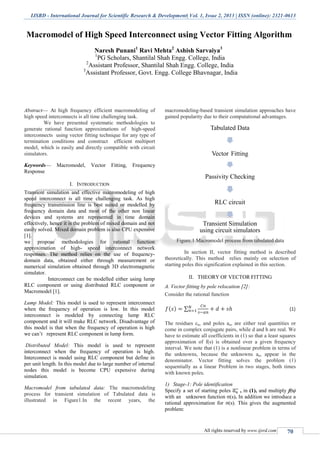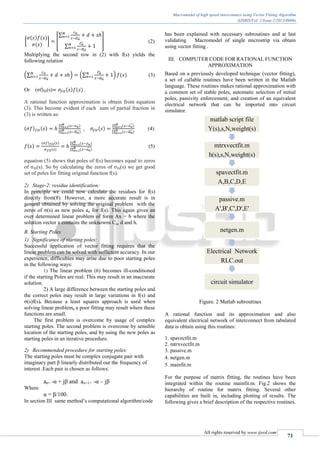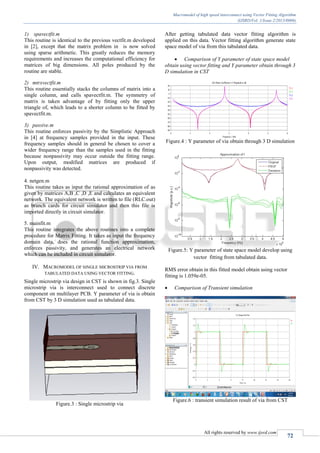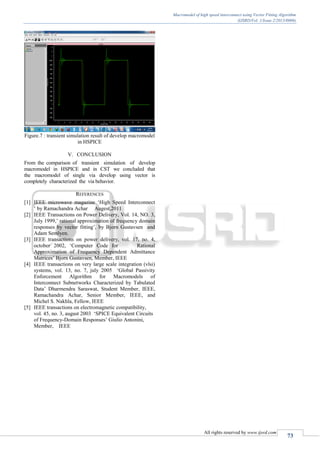Nächste SlideShare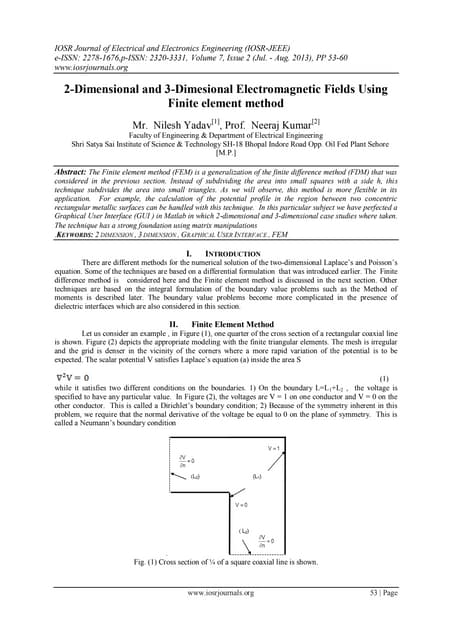2-Dimensional and 3-Dimesional Electromagnetic Fields Using Finite element me...
1 von 42-Dimensional and 3-Dimesional Electromagnetic Fields Using Finite element me...IOSR JournalsA New Approach to Linear Estimation Problem in Multiuser Massive MIMO SystemsRadita Apriana2010 - Stapelberg, Krzesinski - Network Re-engineering using Successive Survi...Dieter StapelbergA Weighted Duality based Formulation of MIMO SystemsIJERA EditorEr4301866870IJERA EditorAn Efficient Clustering Method for Aggregation on Data FragmentsIJMER

### Macromodel of High Speed Interconnect using Vector Fitting Algorithm

1. IJSRD - International Journal for Scientific Research & Development| Vol. 1, Issue 2, 2013 | ISSN (online): 2321-0613 All rights reserved by www.ijsrd.com 70 Macromodel of High Speed Interconnect using Vector Fitting Algorithm Naresh Punani1 Ravi Mehta2 Ashish Sarvaiya3 1 PG Scholars, Shantilal Shah Engg. College, India 2 Assistant Professor, Shantilal Shah Engg. College, India 3 Assistant Professor, Govt. Engg. College Bhavnagar, India Abstract— At high frequency efficient macromodeling of high speed interconnects is all time challenging task. We have presented systematic methodologies to generate rational function approximations of high-speed interconnects using vector fitting technique for any type of termination conditions and construct efficient multiport model, which is easily and directly compatible with circuit simulators. Keywords— Macromodel, Vector Fitting, Frequency Response I. INTRODUCTION Transient simulation and effective macromodeling of high speed interconnect is all time challenging task. As high frequency transmission line is best suited or modelled by frequency domain data and most of the other non linear devices and systems are represented in time domain effectively, hence it is the problem of mixed domain and not easily solved. Mixed domain problem is also CPU expensive . we propose methodologies for rational function approximation of high- speed interconnect network responses. The method relies on the use of frequency- domain data, obtained either through measurement or numerical simulation obtained through 3D electromagnetic simulator. Interconnect can be modelled either using lump RLC component or using distributed RLC component or Macromodel . Lump Model: This model is used to represent interconnect when the frequency of operation is low. In this model interconnect is modeled by connecting lump RLC component and it will make RLC network. Disadvantage of this model is that when the frequency of operation is high we can’t represent RLC component in lump form. Distributed Model: This model is used to represent interconnect when the frequency of operation is high. Interconnect is model using RLC component but define in per unit length. In this model due to large number of internal nodes this model is become CPU expensive during simulation. Macromodel from tabulated data: The macromodeling process for transient simulation of Tabulated data is illustrated in Figure1.In the recent years, the macromodeling-based transient simulation approaches have gained popularity due to their computational advantages. Figure.1 Macromodel process from tabulated data In section II, vector fitting method is described theoretically. This method relies mainly on selection of starting poles this signification explained in this section. II. THEORY OF VECTOR FITTING A. Vector fitting by pole relocation : Consider the rational function ( ) ∑ (1) The residues cn, and poles an, are either real quantities or come in complex conjugate pairs, while d and h are real. We have to estimate all coefficients in (1) so that a least squares approximation of f(s) is obtained over a given frequency interval. We note that (1) is a nonlinear problem in terms of the unknowns, because the unknowns an, appear in the denominator. Vector fitting solves the problem (1) sequentially as a linear Problem in two stages, both times with known poles. 1) Stage-1: Pole identification Specify a set of starting poles ̅̅̅ , in (1), and multiply f(s) with an unknown function σ(s). In addition we introduce a rational approximation for σ(s). This gives the augmented problem: Tabulated Data Vector Fitting Passivity Checking RLC circuit Transient Simulation using circuit simulators
2. Macromodel of high speed interconnect using Vector Fitting Algorithm (IJSRD/Vol. 1/Issue 2/2013/0006) All rights reserved by www.ijsrd.com 71 [ ( ) ( ) ( ) ] [ ∑ ̅̅̅̅ ∑ ̃ ̅̅̅̅ ] (2) Multiplying the second row in (2) with f(s) yields the following relation (∑ ̅̅̅̅ ) (∑ ̃ ̅̅̅̅ ) ( ) (3) Or (σf)fit(s) ( ) ( ) . A rational function approximation is obtain from equation (3). This become evident if each sum of partial fraction in (3) is written as: ( ) ( ) ∏ ( ) ∏ ( ̅̅̅̅) , ( ) ∏ ( )̃ ∏ ( ̃ ) (4) ( ) ( ) ( ) ( ) ∏ ( ) ∏ ( )̃ (5) equation (5) shows that poles of f(s) becomes equal to zeros of σfit(s). So by calculating the zeros of σfit(s) we get good set of poles for fitting original function f(s). 2) Stage-2: residue identification: In principle we could now calculate the residues for f(s) directly from(8). However, a more accurate result is in general obtained by solving the original problem with the zeros of σ(s) as new poles an for f(s). This again gives an over determined linear problem of form Ax = b where the solution vector x contains the unknowns Cn, d and h. B. Starting Poles 1) Significance of starting poles: Successful application of vector fitting requires that the linear problem can be solved with sufficient accuracy. In our experience, difficulties may arise due to poor starting poles in the following ways: 1) The linear problem (6) becomes ill-conditioned if the starting Poles are real. This may result in an inaccurate solution. 2) A large difference between the starting poles and the correct poles may result in large variations in f(s) and σ(s)f(s). Because a least squares approach is used when solving linear problem, a poor fitting may result where these functions are small. The first problem is overcome by usage of complex starting poles. The second problem is overcome by sensible location of the starting poles, and by using the new poles as starting poles in an iterative procedure. 2) Recommended procedure for starting poles: The starting poles must be complex conjugate pair with imaginary part β linearly distributed our the frequency of interest .Each pair is chosen as follows: an= -α + jβ and an+1= -α – jβ Where α = β/100. In section III same method’s computational algorithm/code has been explained with necessary subroutines and at last validating Macromodel of single microstrip via obtain using vector fitting . III. COMPUTER CODE FOR RATIONAL FUNCTION APPROXIMATION Based on a previously developed technique (vector fitting), a set of callable routines have been written in the Matlab language. These routines makes rational approximation with a common set of stable poles, automatic selection of initial poles, passivity enforcement, and creation of an equivalent electrical network that can be imported into circuit simulator. Figure. 2 Matlab subroutines A rational function and its approximation and also equivalent electrical network of interconnect from tabulated data is obtain using this routines: 1. spavectfit.m 2. mtrxvectfit.m 3. passive.m 4. netgen.m 5. mainfit.m For the purpose of matrix fitting, the routines have been integrated within the routine mainfit.m. Fig.2 shows the hierarchy of routine for matrix fitting. Several other capabilities are built in, including plotting of results. The following gives a brief description of the respective routines. matlab script file Y(s),s,N,weight(s) mtrxvectfit.m h(s),s,N,weight(s) spavectfit.m A,B,C,D,E passive.m A',B',C',D',E' netgen.m Electrical Network RLC.out circuit simulator
3. Macromodel of high speed interconnect using Vector Fitting Algorithm (IJSRD/Vol. 1/Issue 2/2013/0006) All rights reserved by www.ijsrd.com 72 1) spavectfit.m This routine is identical to the previous vectfit.m developed in , except that the matrix problem in is now solved using sparse arithmetic. This greatly reduces the memory requirements and increases the computational efficiency for matrices of big dimensions. All poles produced by the routine are stable. 2) mtrxvectfit.m This routine essentially stacks the columns of matrix into a single column, and calls spavectfit.m. The symmetry of matrix is taken advantage of by fitting only the upper triangle of, which leads to a shorter column to be fitted by spavectfit.m. 3) passive.m This routine enforces passivity by the Simplistic Approach in  at frequency samples provided in the input. These frequency samples should in general be chosen to cover a wider frequency range than the samples used in the fitting because nonpassivity may occur outside the fitting range. Upon output, modified matrices are produced if nonpassivity was detected. 4. netgen.m This routine takes as input the rational approximation of as given by matrices A,B ,C ,D ,E and calculates an equivalent network. The equivalent network is written to file (RLC.out) as branch cards for circuit simulator and then this file is imported directly in circuit simulator. 5. mainfit.m This routine integrates the above routines into a complete procedure for Matrix Fitting. It takes as input the frequency domain data, does the rational function approximation, enforces passivity, and generates an electrical network which can be included in circuit simulator. IV. MACROMODEL OF SINGLE MICROSTRIP VIA FROM TABULATED DATA USING VECTOR FITTING. Single microstrip via design in CST is shown in fig.3. Single microstrip via is interconnect used to connect discrete component on multilayer PCB. Y parameter of via is obtain from CST by 3 D simulation used as tabulated data. Figure.3 : Single microstrip via After getting tabulated data vector fitting algorithm is applied on this data. Vector fitting algorithm generate state space model of via from this tabulated data.  Comparison of Y parameter of state space model obtain using vector fitting and Y parameter obtain through 3 D simulation in CST Figure.4 : Y parameter of via obtain through 3 D simulation Figure.5: Y parameter of state space model develop using vector fitting from tabulated data. RMS error obtain in this fitted model obtain using vector fitting is 1.059e-05.  Comparison of Transient simulation Figure.6 : transient simulation result of via from CST
4. Macromodel of high speed interconnect using Vector Fitting Algorithm (IJSRD/Vol. 1/Issue 2/2013/0006) All rights reserved by www.ijsrd.com 73 Figure.7 : transient simulation result of develop macromodel in HSPICE V. CONCLUSION From the comparison of transient simulation of develop macromodel in HSPICE and in CST we concluded that the macromodel of single via develop using vector is completely characterized the via behavior. REFERENCES  IEEE microwave magazine ‘High Speed Interconnect ‘ by Ramachandra Achar Augest,2011  IEEE Transactions on Power Delivery, Vol. 14, NO. 3, July 1999,’ rational approximation of frequency domain responses by vector fitting’, by Bjorn Gustavsen and Adam Semlyen.  IEEE transactions on power delivery, vol. 17, no. 4, october 2002, ‘Computer Code for Rational Approximation of Frequency Dependent Admittance Matrices’ Bjorn Gustavsen, Member, IEEE  IEEE transactions on very large scale integration (vlsi) systems, vol. 13, no. 7, july 2005 ‘Global Passivity Enforcement Algorithm for Macromodels of Interconnect Subnetworks Characterized by Tabulated Data’ Dharmendra Saraswat, Student Member, IEEE, Ramachandra Achar, Senior Member, IEEE, and Michel S. Nakhla, Fellow, IEEE  IEEE transactions on electromagnetic compatibility, vol. 45, no. 3, august 2003 ‘SPICE Equivalent Circuits of Frequency-Domain Responses’ Giulio Antonini, Member, IEEE